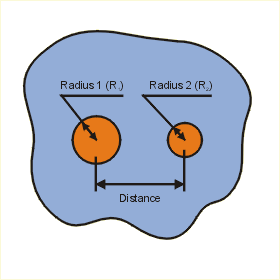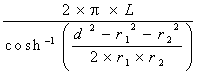Close

This page calculates the temperature of one isothermal (constant temperature) embedded cylinder (T1) and a second cylinder at known temperature (T2) and heat (Q) conducted from cylinder 1 to 2. The two cylinders are embedded in an infinite medium of conductivity (k).The plate temperature T1 is calculated as:

T1 = T2 + q / (k x sf)

Where T2 is the isothermal temperature applied to cylinder 2, q is the heat flow, sf is the shape factor, and k is the conductivity of the medium surrounding the cylinders. Heat flows from cylinder 1 to 2. That is, cylinder 1 is hotter than cylinder 2

The shape factor is only calculated when the cylinder length is much larger than the cylinder radii (at least 10 times larger) and the length of the embedded cylinders is much larger than the distance between them. In this case, the shape factor is calculated as:

sf =Where r1 is the cylinder 1 radius, r2 is the cylinder 2 radius, L is the cylinder length (both cylinders are assumed to have the same length), and d is the distance between the cylinders.

References

Holman, J.P., Heat Transfer, 7th ed., McGraw Hill Book Company, New York, 1990. p 74 - 83